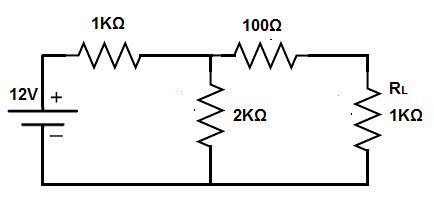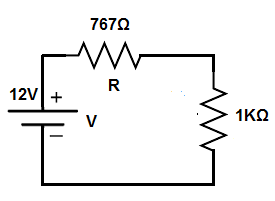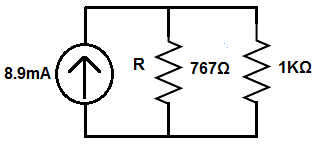﻿ Norton's Theorem- Explained ﻿# Norton's TheoremIn this article, we explain norton's theorem.

Norton's theorem states that any combination of power sources and resistors can be replaced with a single current source in parallel with a single resistor.

Norton's theorem, thus, greatly reduces and simplifies a circuit.

In the end, norton's theorem produces a single current source with a single resistance in parallel, along with the load. Like the thevenin equivalent, the norton equivalent does not take into account the resistance of the load (the output the circuit is powering). Therefore, at the end is a circuit that produces the norton current source, norton resistance, and a the load resistance.

Therefore, in the end, a current divider circuit exists between the norton equivalent resistance and the load resistance, and we can calculate how much current each gets through the current division formula.

The great thing about norton's theorem is the simplicity it creates. Basically, norton's equivalent looks at the entire circuit except the load of the circuit. It then simplifies all the power sources into a single current source and all resistors into a single equivalent resistor (in parallel with the current source). At the end, we just have the single current source, in parallel with a resistor, which is in parallel with the load (resistance). It forms a very simple current division circuit between the single equivalent resistor and the load resistance.

In thevenin's theorem, the circuit is reduced to a single voltage source in series with a single resistor, along with the load resistance, forming a voltage divider circuit.

So let's now go over an example circuit, so that you can see how norton's theorem works.

### Example of Norton's Theorem

So, below, we have a circuit that we will break down.So this circuit is a typical circuit that has a voltage source and several resistors.

In order to produce the equivalent norton resistance, we have to simplify all of the resistors into an equivalent resistance, excluding the load (load resistance).

To do this, we find the resistance of the 1KΩ and the 2KΩ resistor. Since they are in parallel, the equivalent resistance is 1KΩ || 2KΩ = (1KΩ)(2KΩ)/(1KΩ + 2KΩ)= 667Ω.

We then add the 100Ω resistor to the 667Ω equivalent resistance. This gives us 767Ω.

So the equivalent norton resistance is 767Ω.

This is shown in the circuit below.So now you see that we have a voltage source and 2 resistors that are in series.

This forms a voltage divider circuit.

The 12V divides up between the 767Ω and the 1KΩ resistor.

Doing the calculations, this yields 5.2V across the 767Ω resistor, since 12V(767Ω)/(767Ω + 1KΩ)= 5.2V and 6.8V across the 1KΩ resistor, since 12V(1KΩ)/(767Ω + 1KΩ)= 6.8V.

To calculate the norton current source, we take the voltage across the load (which is 6.8V) and divide it by the norton equivalent resistance (which is 767Ω). This is simply ohm's law, I= V/R= 6.8V/767Ω= 8.9mA.

This is shown in the circuit below.So now this is norton's equivalent circuit.

You can see how the norton's equivalent circuit forms a current divider circuit between the norton equivalent resistance and the resistance of the load.

The norton current source is 8.9mA. So this 8.9mA divides into the 2 resistors.

Using the current division formula, we can calculate how much each branch gets.

The current division formula is, IS= RT/RX, where IS is the current from the power source, RT is the equivalent resistances of the branches in a current divider, and RX is the specific resistance of the branch you are calculating the current flow through.

So IS is 8.9mA, because that's the current flowing from the currnet source.

RT is the equivalent resistance of all the branches of the current divider circuit. Being that there is 2 resistances in the current divider circuit, 767Ω and 1KΩ, the equivalent resistance is 767Ω || 1KΩ. Doing the math, the equivalent resistance is, 767 || 1KΩ = (767Ω)(1KΩ)/(767Ω+1KΩ)= 434Ω.

RX is the specific resistance of the branch you are calculating the current for.

Therefore, the current through the 767Ω resistor is, (8.9mA)(434Ω)/(767Ω)= 5.04mA.

The current through the 1KΩ is, (8.9mA)(434Ω)/(1KΩ)= 3.86mA.

Therefore, through norton's equivalent, a current divider circuit is formed, which we can easily calculate currents through various branches of the circuit.

So norton's theorem allows you to take any circuit with any number of power sources and reduce it to a single current source in parallel with a single resistance, along with the load. This forms a simple current divider circuit, that allows for easy analysis of the circuit.

Related Resources

HTML Comment Box is loading comments...

﻿